# Averaging filters

Author: Wolfgang Scherr
Date: 10th February 2021

(c) Carinthia University of Applied Sciences

SystemC-AMS models created with Coside.

# Moving average filters

This are filter structures, which calculates the average of a defined amount of input samples continuously. Which each new sample, the furthest 'away' sample is removed again:The difference equation for a 5-tap moving averaging filter is:

$$y[n] = \frac{1}{5}(x[n] + x[n-1] + x[n-2] + x[n-3] + x[n-4])$$

$$x[n]$$ is the actual sample, $$x[n-1]$$ is the last sample, $$x[n-2]$$ is the next-to-last sample and so on.
In a general form, the equation looks for a filter with length N like:

$$y[n] = \frac{1}{N}\sum_{k=0}^{N-1}x[n-k]$$

As this filter levels out fast changes, it has a low-pass characteristics, let's check if this is true.

So we do the z-transform: $$x[n-k]\rightarrow X[z]z^{-k}$$ and get:

$$Y[z] = \frac{1}{N}X[z]\sum_{k=0}^{N-1}z^{-k}$$

Finally, we can write the transfer function as:

$$H[z] = \frac{Y[z]}{X[z]} = \frac{1}{N}\sum_{k=0}^{N-1}z^{-k}$$

Looking at the frequency behavior at the unit cycle $$z = e^{j\omega}$$ (z transform), using $$\sum_{k=0}^{n}r^k = \frac{1-r^{n+1}}{1-r}$$ (finite sum of the geometric series) and using $$e^{j a} = cos(a) - j sin(a)$$ (Euler's formula) we get:

$$H[e^{j\omega}] = \frac{1}{N}\sum_{k=0}^{N-1}e^{-j\omega k} = \frac{1}{N}\frac{1-e^{-j\omega N}}{1-e^{-j\omega}} = \frac{1}{N}\frac{e^{-\frac{j\omega N}{2}}\left(e^{\frac{j\omega N}{2}}-e^{-\frac{j\omega N}{2}}\right)} {e^{-\frac{j\omega}{2}}\left(e^{\frac{j\omega}{2}}-e^{-\frac{j\omega}{2}}\right)} = \frac{1}{N}\frac{sin \frac{\omega N}{2}}{sin \frac{\omega}{2}}e^{-\frac{j\omega}{2}(N-1)}$$

# Frequency response plot for averaging filters

We plot the absolute transfer function for several length N from 0 to half the normalised sample frequency $$f_s$$.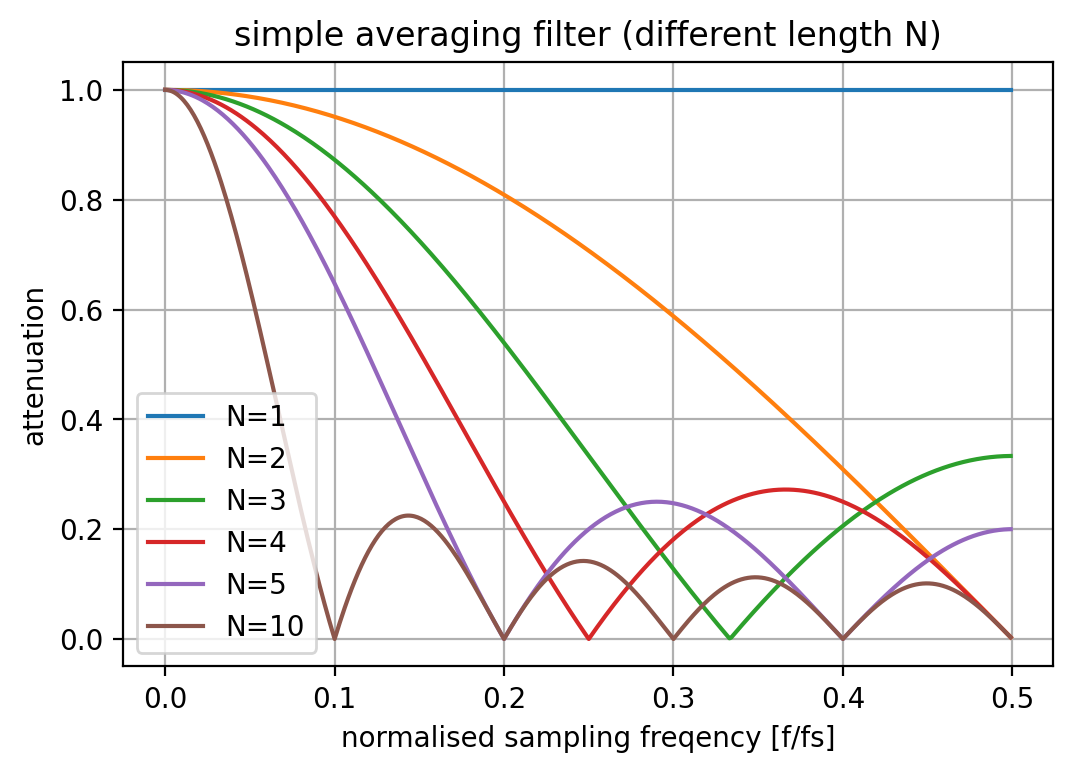# Check frequency response by simulation

We will use SystemC-AMS using the TDF MoC for transient noise and AC simulation.First, we send a noise signal into the filter (with constant power up to $$f_s/2$$):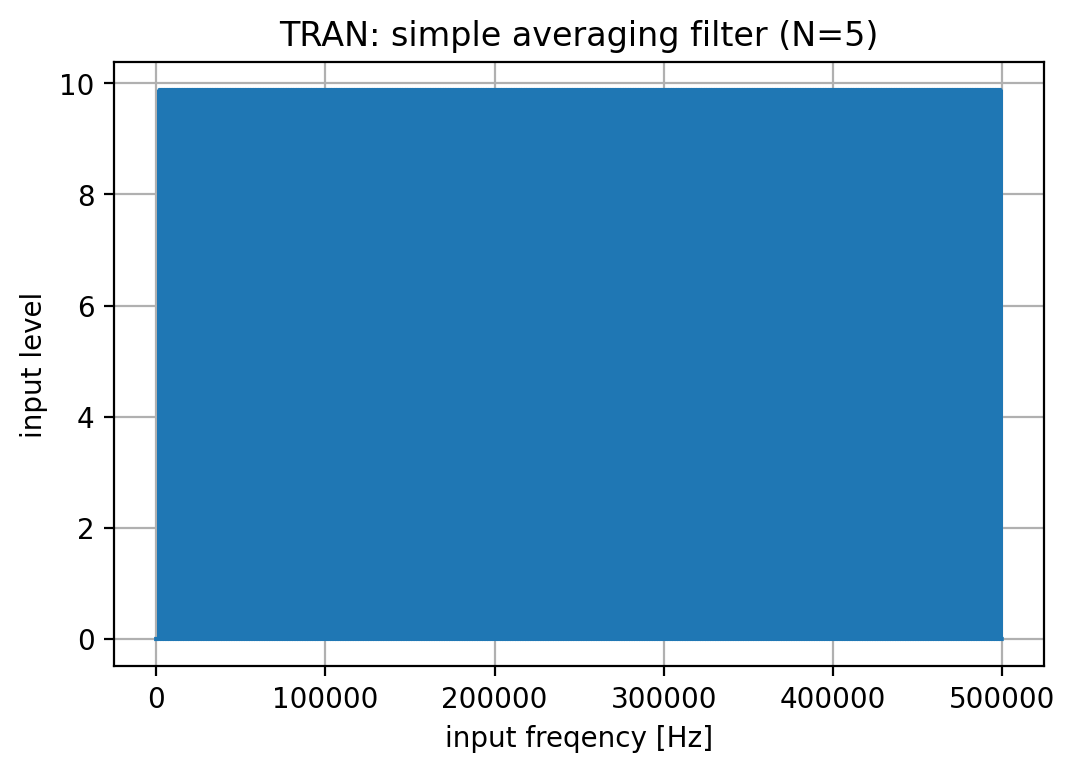Then we look at the FFT of the transient simulation result on its output: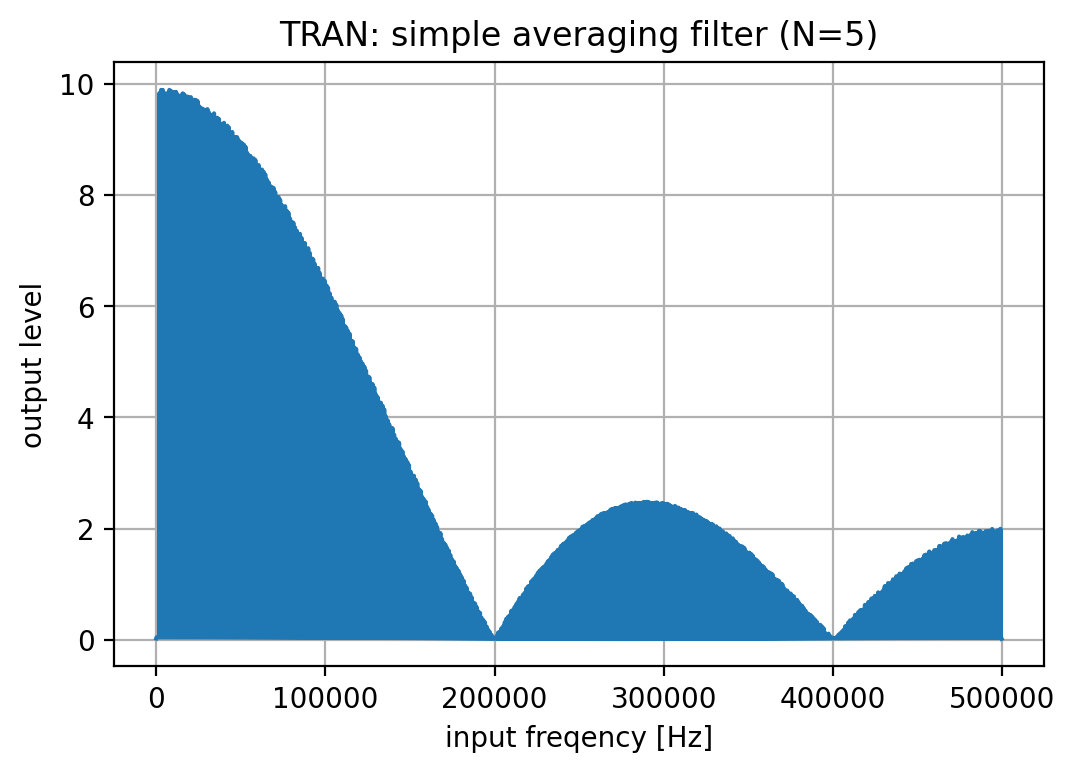Finally, we do an AC simulation and look at the result (as absolute value):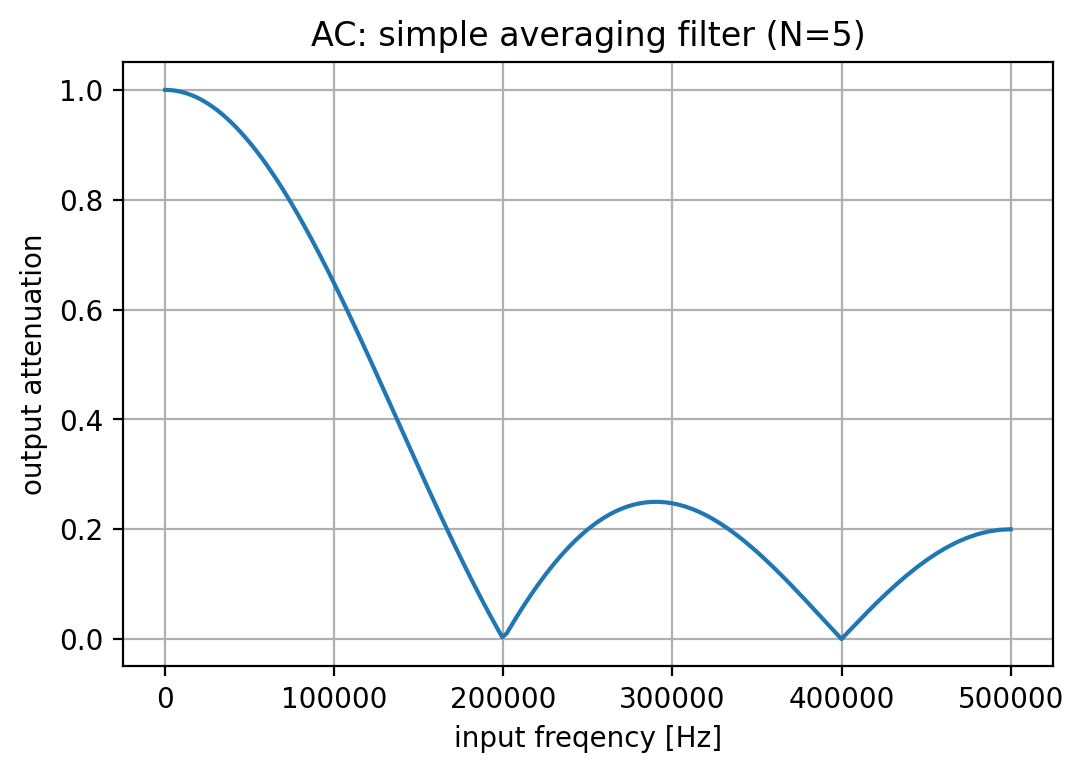# Area and power consumption (qualitative)

The requirement for such a simple moving average filter of length N, assuming a single-bit input is:

• Area: (N-1) flip-flops, (N-2) full adder
• Power: flip-flops (N-1) @ fs, full adder log2(N-2) @ fs

For our example with N=5, 4 FF and 3 full adder are required.
For an averager with N=16, this goes up to 15 FF and 14 full adder.

The question is now, is this the best we can do?

# Improved implementation of averaging filters

The simple averaging filter delivers the moving average result with the same rate as given by its input. But when using this filter for decimation, this is not really needed. So by some translations we can show, that there is a more efficient way to achieve the same result.

The intermediate steps lead to the very same behavior as the simple averager, we spare the simulation for these ones here.

In the first step, we create an integrator where we add the actual and subtract the Nth sample using a N-stage differentiator. The integrator needs only log2(N) FF and log2(N) half adders. So not really better than before...It seems that the next step does not really make sense, as it increases the bit width for the differentiator. So it looks we made it even worse, but just wait a bit. It will get much better in a moment...Now we introduce the decimation between these two stages. This means that the N-stage differentiator can be reduced to a single stage running at the frequency of $$f_s/N$$:The requirement for such an average filter of length N, assuming a single-bit input is now:

• Power: flip-flops log(N) @ fs, log2(N) @ fs/N, half adder log2(N) @ fs, full adder log2(N) @ fs/N

For our example with N=5: 6 FF, 3 half adder, 3 full adder are required.
For an averager with N=16: 8 FF, 4 half adder, 4 full adder is more efficient than the simple solution!
I spare the power estimation here, as I believe you see the advantage on that one by yourself.

This brings us to the Hogenauer filter, also called CIC filter, as described e.g. here by Matthew P. Donadio.

# Verify the new filter

We do again a transient simulation with a band-limited noise on the input to check the frequency response.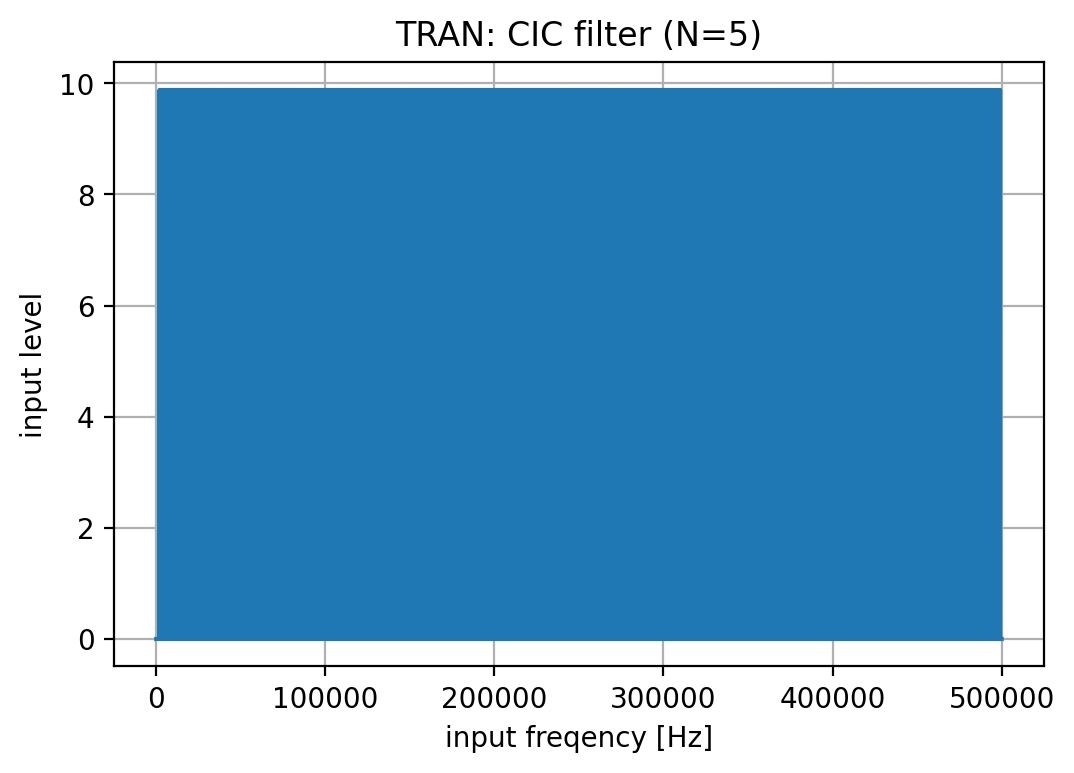When we look at the FFT of the transient simulation result on its output, we get 5 times less samples on the output for the FFT. The data we analyse is similar to an zero-order hold with 5*fs connected after the decimation.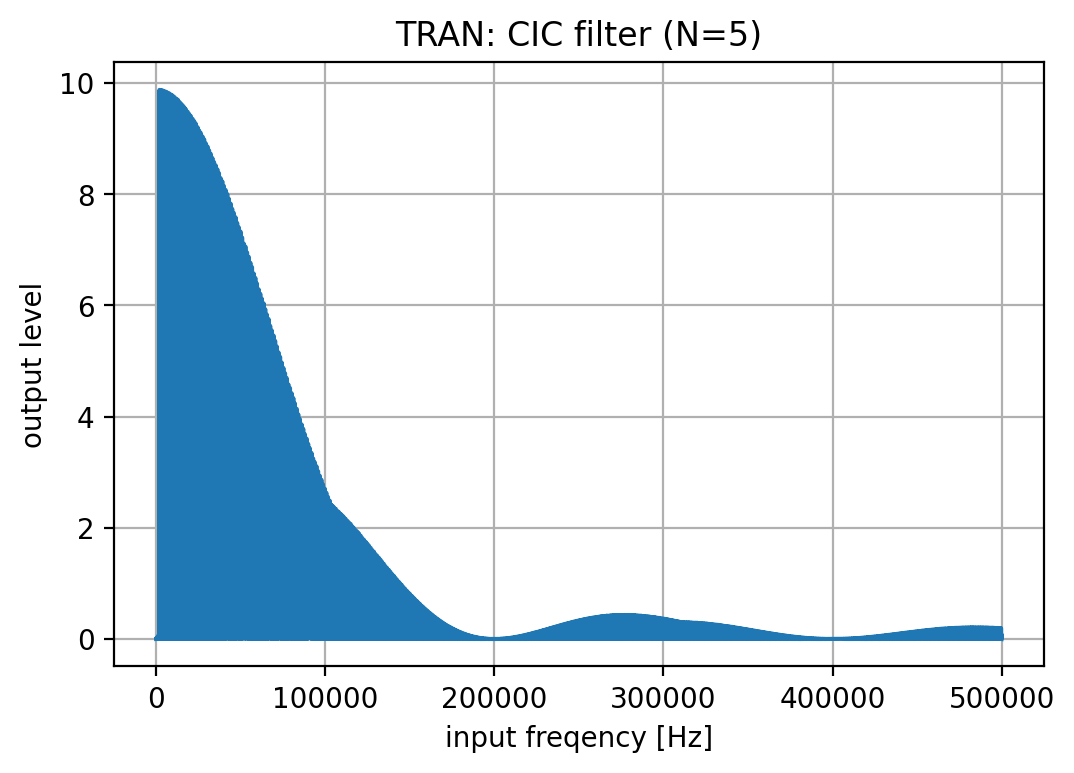Be aware, the output spectrum basically 'ends' at a five times lower frequency, so to say. Everything above the new fs/2 = 100kHz is folded back down into the signal band. Furthermore, all notches at 200kHz and 400kHz of the upper sampling frequency fold back to 0 Hz on the lower (output) sampling frequency.

It is better to see if we look at the decimated output data at fs=200kHz. here we can see the original frequency response of the averaging filter within 0-500kHz folded into 0-100kHz.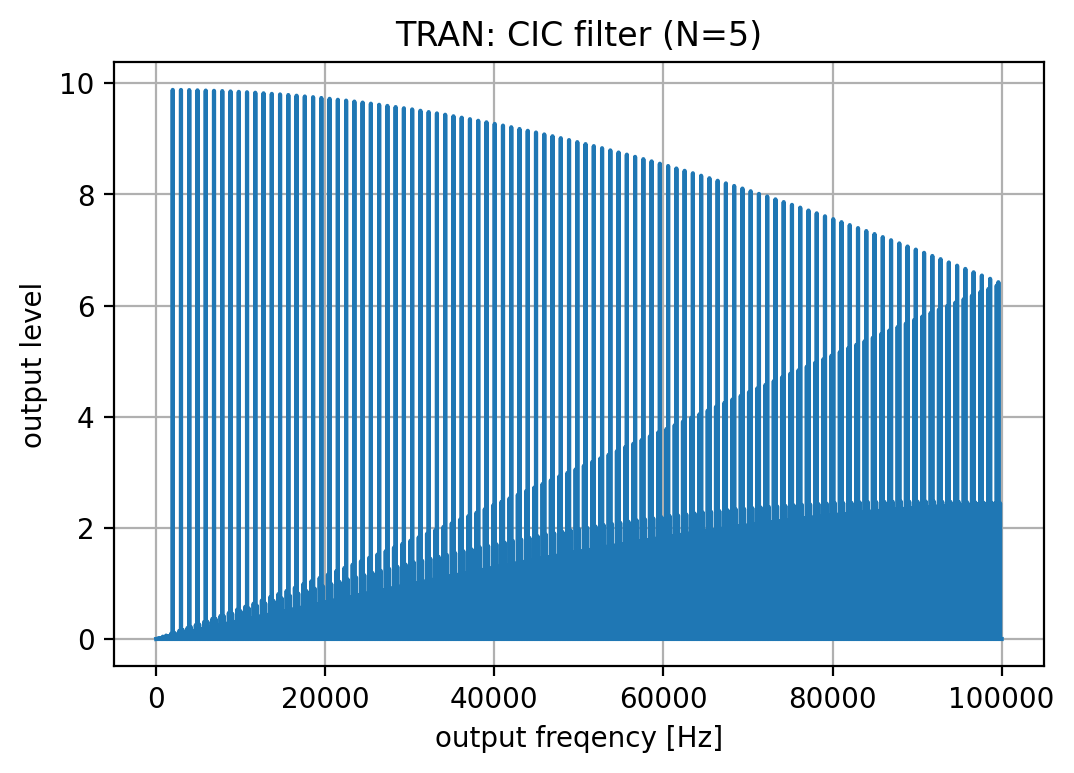It also demonstrates that a 1.O. averaging filter is not really good for decimation, you should always consider higher order filters.

# Conclusion

This report gave a quick introduction to averaging filters, from a very basic design to broadly used CIC filters. The improvement in power consumption and area was briefly illustrated as well in a qualitative sense.

Here the final result in a compact table:The report finally passes on to a nice paper explaining CIC filters in more depth.